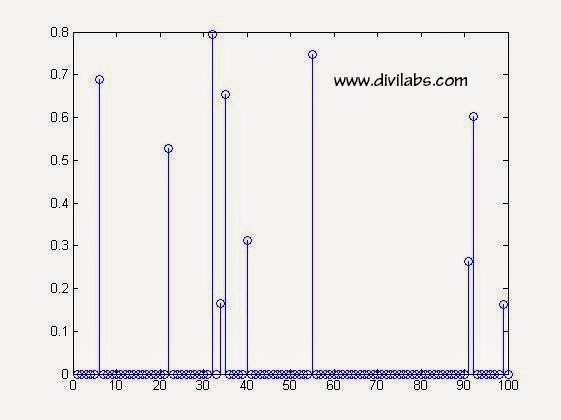# Generate a Random Sparse Signal Vector Using Randperm Function in MATLAB

Yes Matlab does have an inbuilt sparse data type & related function, which can be used very easily to generate a random sparse Vector. ( See :Creating & Using Sparse Matrix & Vectors in MATLAB - Concepts & Examples )
But there are other ways also in which you can generate it. One of them is through randperm() function of matlab.
To generate in this way it involves a little trick & some understanding upon how the Matlab's variable/vector index works & how randperm function works.

MATLAB Code for Random Sparse Signal Vector Using Randperm Function Will look Like this:

clc
clear all
close all
n=100;  %generation 100x1 vector sparse
k=10;
%Out of 'n' elements 'k' are non zero
xorg=zeros(n,1);
%initialization of the sparse vector as a zero vector 'xorg'
idx=randperm(n);  %we are calculating the index of first 'k' non zero elements through this step
xorg(idx(1:k))=rand(k,1); %take first 'k' elements of 'idx' & fill those index with a random value
stem(xorg) %plot the sparse vector thus generated

NOTE: # initializing by xorg=zeros(n,1); is necessary since if you are not doing this, you will generate x(org) in each run, with a different dimension.
# instead of rand(k,1) one can use randn(k,1) also.

Sample Run Of the Above MATLAB's Code:Random Sparse Signal Vector Using Randperm Function in MATLAB
How the Code Works?

** xorg=zeros(n,1); -> This command generate a 'n x 1' column vector of all elements being zero.

** idx=randperm(n); -> This command will generate a '1 x n' vector in which the elements are distributed randomly between 1 to n  (including this also). There is no repetition of any number between 1 to n, i.e., every element will be unique in this vector.

e.g., in the above it is,
idx = 99    32    40    22    34    92    91    35     6    55     3    96    68    16    69 & so on

** xorg(idx(1:k))=rand(k,1); -> idx(1:k) will select first 'k' elements from 'idx' here in this example it will be  99    32    40    22    34    92    91    35     6    55 & take them to select those index of 'xorg'. Then set those index value of 'xorg' to some random generated value ranging from 0 to 1 (since we are using rand( ) function & using  stem(xorg); -> plot the generated sparse signal

You can verify that index number 99    32    40    22    34    92    91    35     6    55 are non zero & others are all zero.
In our execution, the result was.
xorg=

0
0
0
0
0
0.689214503140008
% 6th index
0
0
0
0
0
0
0
0
0
0
0
0
0
0
0
0.528533135506213
% 22nd index
0
0
0
0
0
0
0
0
0
0.794284540683907
%32nd index
0
0.165648729499781
%34th index
0.654079098476782 %35th index
0
0
0
0
0.311215042044805
%40th index
0
0
0
0
0
0
0
0
0
0
0
0
0
0
0.748151592823710
%55th index
0
0
0
0
0
0
0
0
0
0
0
0
0
0
0
0
0
0
0
0
0
0
0
0
0
0
0
0
0
0
0
0
0
0
0
0.262971284540144
%91th index
0.601981941401637
%92nd index
0
0
0
0
0
0
0.162182308193243
%99th index
0